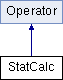# StatCalc Class Reference [Evolutionary Framework, Population]

Statistics calculation class. More...

`#include <StatCalc.h>`

Inheritance diagram for StatCalc:List of all members.

## Public Member Functions

bool operate (StateP)
perform the designated operation
bool operate (const std::vector< IndividualP > &)
Calculate basic fitness statistics on given individual pool. Add new statistic for each measure (min, max, avg, dev, time, size, low, high).
void registerParameters (StateP)
Register parameters with the Registry. Called before Operator::initialize.
bool initialize (StateP)
Perform initialization. Called before Operator::operate. By default, if the return value is false, the operator will not be used!
bool update (std::vector< double >)
void log (int generation=-1)
std::vector< double > getStats (int generation=-1)
void output (uint step)
void copyStats (StatCalcP)
double getFitnessMin (int generation=-1)
double getFitnessMax (int generation=-1)
uint increaseEvaluations (uint eval=1)
void setEvaluations (uint eval)
uint getEvaluations ()
double getLowestFitness ()
double getHighestFitness ()

## Detailed Description

Statistics calculation class.

Definition at line 19 of file StatCalc.h.

## Member Function Documentation

 void StatCalc::copyStats ( StatCalcP stats )

Copy latest statistics from another Stat object.

Definition at line 132 of file StatCalc.cpp.

 uint StatCalc::getEvaluations ( ) ` [inline]`

get total number of fitness evaluations

Definition at line 73 of file StatCalc.h.

 double StatCalc::getFitnessMax ( int generation = `-1` )

Get maximum fitness value for a given generation.

Definition at line 59 of file StatCalc.cpp.

 double StatCalc::getFitnessMin ( int generation = `-1` )

Get minimum fitness value for a given generation.

Definition at line 48 of file StatCalc.cpp.

 double StatCalc::getHighestFitness ( ) ` [inline]`

get highest ever fitness vale

Definition at line 85 of file StatCalc.h.

 double StatCalc::getLowestFitness ( ) ` [inline]`

get lowest ever fitness vale

Definition at line 79 of file StatCalc.h.

 std::vector< double > StatCalc::getStats ( int gen = `-1` )

Get statistic values for a given generation.

Definition at line 205 of file StatCalc.cpp.

 uint StatCalc::increaseEvaluations ( uint eval = `1` ) ` [inline]`

increase and return total number of fitness evaluations

Definition at line 58 of file StatCalc.h.

 bool StatCalc::initialize ( StateP ) ` [virtual]`

Perform initialization. Called before Operator::operate. By default, if the return value is false, the operator will not be used!

Returns:
initialization success

Reimplemented from Operator.

Definition at line 19 of file StatCalc.cpp.

 void StatCalc::log ( int generation = `-1` )

Log statistics.

Definition at line 228 of file StatCalc.cpp.

 void StatCalc::setEvaluations ( uint eval ) ` [inline]`

set total number of fitness evaluations

Definition at line 67 of file StatCalc.h.

 bool StatCalc::update ( std::vector< double > stats )

Merge current statistics with additional individual set's statistics.

Definition at line 153 of file StatCalc.cpp.

The documentation for this class was generated from the following files: